# Karnaugh Map (K-map)

Definition: Karnaugh Map usually abbreviated as K-map is a systematic approach used for simplifying Boolean expressions or logic functions. It is majorly used method for minimizing the Boolean expressions. K map is basically known to be a different method for the representation of truth table.

The Karnaugh map (K map) according to the variables involved can be either 1, 2, 3 or 4 variables. The number of cells for K-maps in case of different variables will be identified by:

2n

where n is the total number of variables.

So, in case of one variable K-map, n will be equal to 1, thus the number of cells in 1 variable K-map will be 2. Similarly, for two variable K-map, n will be 2, hence a number of cells, in this case, will be 4. Also, for 3 variable K-map, the number of cells will be 8 and for n equals to 4, the number of cells will be 16.

In this way, we can say that the number of variables decides the number of cells in the K-map.

Here in this article, we will have a discussion on the rules to draw a K-map, the assigning of variables to the cells and 3, 4 variables Karnaugh map.

### Rules to draw Karnaugh Map

We know that K-map is used for simplification of Boolean expressions. However, some rules are associated whenever a K-map is plotted. The rules are given below:

• In K-map while adding binary terms according to the variables assigned, no 2 variables of adjacent columns can be changed simultaneously.

Have a look at the example of 3 variable K-map shown below:Here, as we can see that in the third column 11 is represented while in 4th column 10 is shown. This is so because as we have written 01 in the 2nd column. And if we write 10 in the 3rd column then simultaneously 2 variables will get changed. As 0 changes to 1 and 1 changes to 0 simultaneously.

This is the reason why m2 is assigned at the rightmost column of the 1st row and m3 is placed before m2. In a similar way, m6 is present after m7.

• When a minterm K-map is designed then, in this condition, 1 is assigned at all those cells for which the output is 1, while 0 is provided at those cells where output is 0. But sometimes for simplicity, these 0’s is omitted and the cells are kept vacant in the case when 0 is required to be filled.

The condition is reversed at the time of designing a maxterm K-map. Here 1 is assigned to the cell in case of 0 at the output and 0 is assigned in case of 1 at the output.

• While assigning the don’t care conditions to the cells of the K-map, ‘X’ or ‘d’ is placed at the respective cell.
• At the time of grouping of bits inside the K-map, the highest priority is provided to a group of 16, further the priority decreases with 8, 4 and 2 bits’ pair.
• The rule of adjacency is highly followed while pairing the bits inside the Karnaugh map. This is the reason; diagonal pairing is not performed in K-map.

Let us move further and understand the 3 and 4 variables K-map by some examples.

## Karnaugh Map for 3 Variables

Suppose that we have to simplify a 3 variable Boolean expression using K map.

We know that the number of cells of the K-map is dependent on the number of variables. So, for 3 variable K map, the number of cells will be 23 i.e., 8.

Let us reduce the function given below using K-map

F (A, B, C) = Σm (0, 1, 2, 4, 7)

The figure below represents the K-map for 3 variables having 8 cells.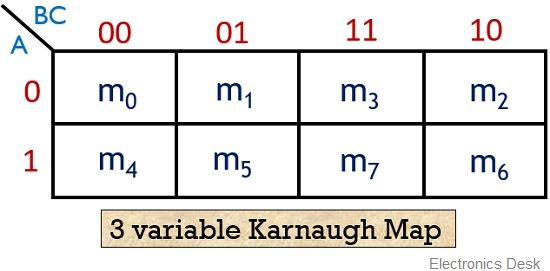As we have already discussed earlier the reason behind the assigning of variables to the Karnaugh map. So, let us now proceed towards filling of bits to the minterm K-map.

The figure below shows the assigning of bits to the K-map: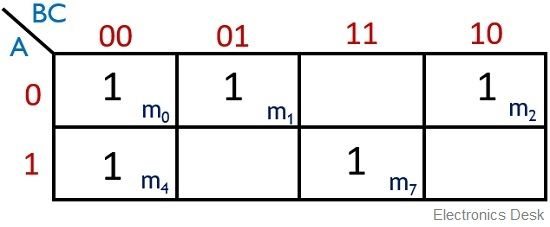Here, we can clearly see that for minterm K-map, 1 is assigned at m0, m1, m2, m4, m7, as given in the function.

So, firstly here we will check the priority, as we can see that neither 16, 8 nor 4 1’s is present in order to perform the grouping. So, we will check for grouping of 2 bits.

The figure below represents the grouping of bits for the above function: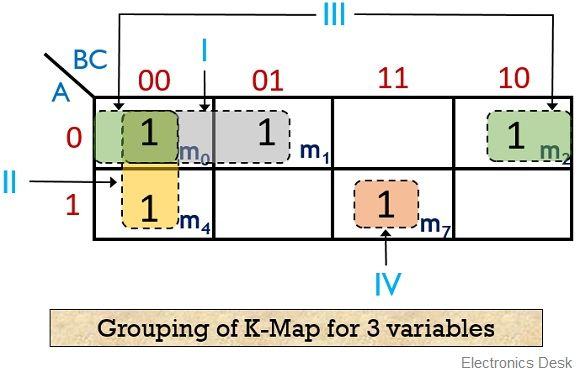Here, we can see that two 1, present at the 1st row of column 00 and 01 are forming pair. Similarly, two 1’s of the first column and rows 0, 1 are forming a pair. Also, the two corners, 1 at the first row is forming a group of 1.

However, still, a single 1 is left that is unable to participate in a grouping as no other 1 is present at its adjacent position. So, this 1 is considered to be as a group of single 1. As represented in the figure given above.

Let us now see how the above function is realized using K-map.

So, the function for all 4 implicants will be: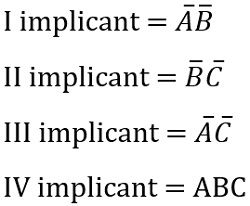So, combinely the realized Boolean expression will be given as:Let us take another example to have a better understanding of the same.

F (A, B, C) = Σm (1, 3, 6, 7)

Let’s have a look at the figure below that represents the placement of bits inside the K-map: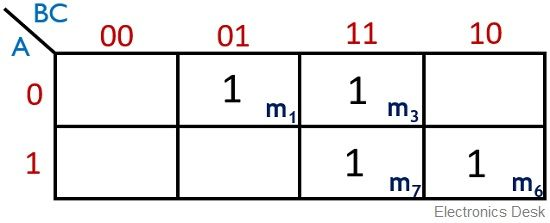The grouping of the bits is shown in the figure below:Here also, neither a group of 8 nor of 4 is forming. Thus 3 implicants can be formed by grouping as shown in the figure above.

Thus the function will be:But a noteworthy point is that here redundancy of bit is generating. This is so because the two 1’s present at BC position is already grouped individually with their adjacent bit. The pairing of two different bits which are separately paired comes under redundancy theorem.

So, in this case, the implicant BC will be ignored. And the realized Boolean expression will be: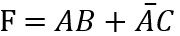Let us now proceed to understand how 4 variable K-map gets realized.

## Karnaugh map for 4 variables

In case of realizing 4 variable K-map, the number of cells will be 24 i.e., 16. At the time of assigning variable to the 4 Χ 4 K-map, again the thing that is to be kept in mind is no two variables can be changed simultaneously.

As we have done by swapping the variables of 3rd and 4th column in the 3 variable K-map.

The figure below represents a Karnaugh map for 4 variables:Suppose the function to be realized is given as:

F (A, B, C, D) = Σm (0, 2, 3, 7, 11, 13, 14, 15)

The figure below represents the bit assigning to the 4 variable K-map: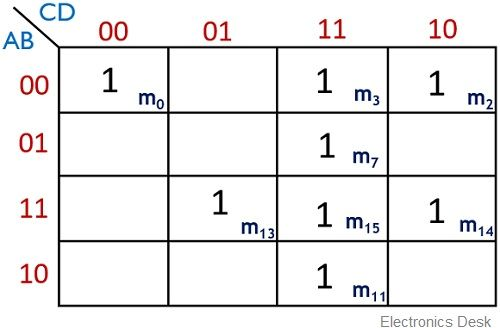Here it is clear from the above figure that 1 is placed at m0, m2, m3, m7, m11, m13, m14, m15.

Now, after the bits get assigned, the grouping is performed. So, again the grouping is done according to their priority.

As we can see that no combination of 8 bits is present in a way to form a group. However, then a group of 4 1’s is formed. Similarly, the two 1’s at the corners of the 1st row are grouped together.

The figure here shows the grouping of bits for the 4 variable functions:So, the above K-map can be realized as: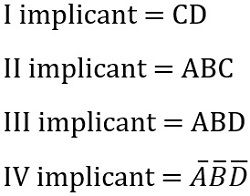Hence the combined realized Boolean expression for the above K-map will be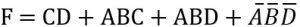### Don’t Care Condition in Karnaugh Map

Till now we have discussed the conditions where the desired output is generated according to some input conditions. But there exist some cases in which the output remains unspecified because of invalid input conditions.

These outputs are denoted as ‘d’ or Χ in the K-map and are known as don’t care condition. Basically whenever these don’t care terms are represented in the K-map then these are utilized in the realization of Boolean expression if required.  Otherwise, these are ignored.

Let us now take an example of a function with 4 variables with don’t care condition.

Suppose the function be:

F (A, B, C, D) = Σm (1, 3, 7, 11, 15) + Σd (0, 2, 4)

So, for the above function the designed K-map is shown below:Here as we can see clearly that at cell position, m0, m2, m4 ‘d’ are placed representing the don’t care condition. However, we have already discussed that in the case of SOP realization these ‘d’ can be considered at the time of grouping.

Thus the grouping inside the K-map can be done in a way shown below: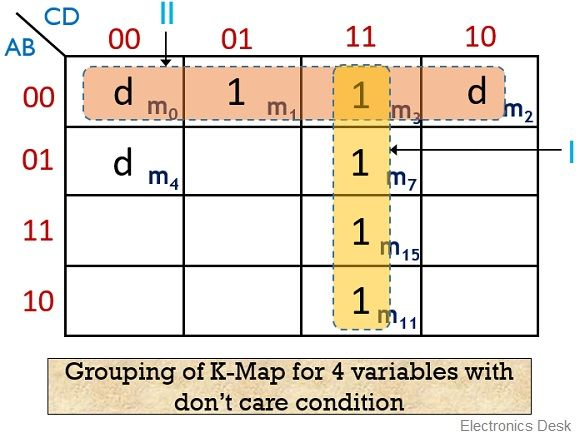Here, by making use of don’t care terms, 2 groups of 4 can be formed. Or we can say 2 quads are formed.

So, the realized function is given asIn this way, various functions are realized using a Karnaugh map technique.